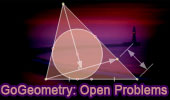# Plane Geometry: Open Problems, High School, CollegeStatic Diagram of problem 902 Dynamic Geometry Problem 902 Post a solution Triangle, Four Squares, Center, Concurrent Lines. GeoGebra, HTML5 Animation for Tablets Static Diagram of problem 901 Dynamic Geometry Problem 901 Post a solution Intersecting Circles, Common External Tangent, Secant, Circumcircle, Concyclic Points, Cyclic Quadrilateral. GeoGebra, HTML5 Animation for Tablets Geometry Problem 894 Post a solution Triangle, Angle, 60 degrees, Incenter, Midpoint, Parallel Lines. GeoGebra, HTML5 Animation for Tablets Geometry Problem 893 Post a solution Triangle, Circumcircle, Incenter, Circle, Tangent, Collinear Points. GeoGebra, HTML5 Animation for Tablets Go to Page: Previous | 1 | 5 | Next

Home | Sitemap | Search | Geometry | Problems | All Problems | Ten Geometry Problems | Visual IndexEmail | by Antonio Gutierrez